Science, Maths & Technology

### Become an OU student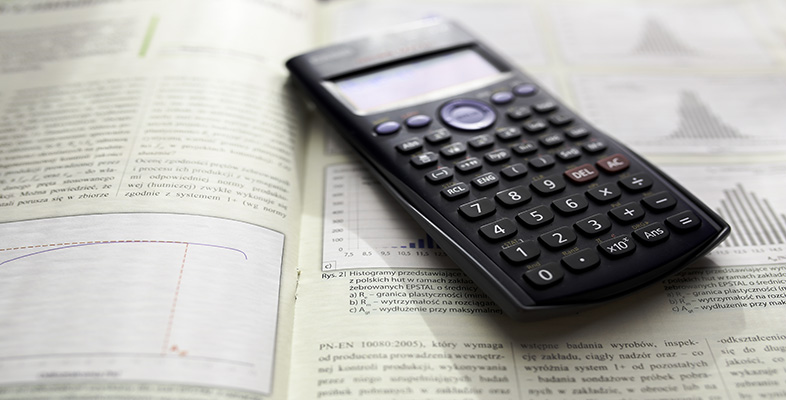Using a scientific calculator

Start this free course now. Just create an account and sign in. Enrol and complete the course for a free statement of participation or digital badge if available.

# 12 Calculator reference guide

## General modes

The calculator can operate in several different modes:

• COMP, which is used for general calculations
• STAT, which is used for statistical calculations
• TABLE, which is used for generating tables of numbers.

COMP is short for ‘computation’, and STAT is short for ‘statistics’.

Comp mode is selected by using the key sequence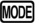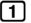(COMP).

## Mathematics modes

There are two different ways in which mathematics can be input to and displayed on the calculator:

• Math mode, in which fractions are entered and displayed in their proper mathematical form – for example,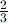– and some irrational numbers are displayed as surds (such as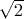) or multiples of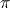(such as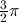).

Math mode is selected by using the key sequence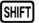(SETUP)(MthIO).

• Linear mode, in which fractions such asare entered and displayed using a linear notation – for example, 2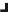3 – and all irrational numbers (such asand) are displayed as decimal approximations.

Linear mode is selected by using the key sequence(SETUP)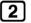(LineIO).

Put simply, an irrational number is one that cannot be expressed as a simple fraction.

You know that you are in Math mode if the word Math is shown near the right-hand side of the top of the calculator display. If this is not shown, you are using Linear mode.

In Math mode, you can force an answer to be displayed as a decimal using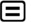, or you can toggle between the mathematical and decimal outputs using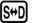.

## Fraction display modes

It is possible to set the calculator so that answers that are top-heavy fractions (such as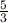) are always displayed as mixed numbers (such as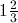).

• To set the calculator to display as mixed numbers, use the key sequence(SETUP)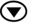(ab/c).
• To set the calculator to display as top-heavy fractions, use the key sequence(SETUP)(d/c).

## Decimal display modes

The calculator can be set to display decimal numbers in various different ways:

• Normal 1 (Norm 1) mode uses scientific notation for any number less than 0.01 but greater than −0.01, and otherwise displays answers to however decimal places they have, subject to the maximum that will fit on the display. This mode is entered using(SETUP)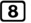(Norm).
• Normal 2 (Norm 2) mode uses scientific notation for any number less than 0.000000001 but greater than −0.000000001, but otherwise displays answers to however many decimal places they have, subject to the maximum that will fit on the display. This mode is entered using(SETUP)(Norm).
• Scientific notation mode (Sci) displays all decimal numbers in scientific notation using a specified number of significant figures. This mode is entered using(SETUP)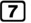(Sci) followed by the number of significant figures required, for example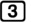. When your calculator is set in this mode, the display indicator SCI is shown.
• Fixed decimal place mode (Fix) displays all decimal numbers to a given number of decimal places (unless scientific notation is needed to fit the result on the display). This mode is entered using(SETUP)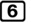(Fix) followed by the number of decimal places required, for example. When your calculator is set in this mode, the display indicator FIX is shown.